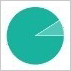# quantize

['kwɒntaɪz]['kwɒntaɪz]• 释义
• 用例
• 更多
• vt. 使量化；数字转换### 释义常用度分布图

点击查看

英英释义
Verb:
1. approximate (a signal varying continuously in amplitude) by one whose amplitude is restricted to a prescribed set of discrete values

2. apply quantum theory to; restrict the number of possible values of (a quantity) or states of (a physical entity or system) so that certain variables can assume only certain discrete magnitudes that are integral multiples of a common factor;

"Quantize gravity"

• 例句
经典引文
• The position was then quantized, that is, expressed as a whole number of unit distances.

出自： F. Smyth

• 近反义词
【近义词】
临近单词